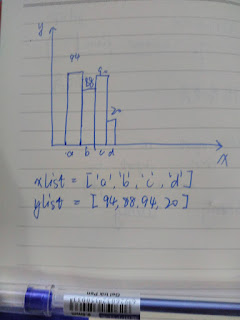# Plot figure which x axis value is strings not numbersHi all, I want to plot a figure like above.

xlist = [94,88,90,20] and

ylist = [‘a’,‘b’,‘c’,‘d’,]

I read bokeh doc but not find proper API to plot a picture like above.Can somebody help? Thanks!

Hi,

Categorical axes are described here in the user's guide:

Thanks,

Bryan

···

On Jul 10, 2016, at 7:58 AM, [email protected] wrote:

Hi all, I want to plot a figure like above.

xlist = [94,88,90,20] and

ylist = ['a','b','c','d',]

I read bokeh doc but not find proper API to plot a picture like above.Can somebody help? Thanks!

--
You received this message because you are subscribed to the Google Groups "Bokeh Discussion - Public" group.
To unsubscribe from this group and stop receiving emails from it, send an email to [email protected].
To post to this group, send email to [email protected].
To view this discussion on the web visit https://groups.google.com/a/continuum.io/d/msgid/bokeh/cec8381a-218a-42cf-bf0b-dfc35b49c486%40continuum.io.

1 Like

Here is a basic code snippet:

from bokeh.charts import Bar, output_file, show, cat
xlist = [‘a’,‘b’,‘c’,‘d’]
ylist = [94,88,90,20]
data = {“x”: xlist, “y”: ylist}
bar = Bar(data, “x”, values=“y”)
output_file(“bar.html”)
show(bar)

Note that the Bar chart will sort the category labels, by default. If you want them to be in the exact order that you passed them in, then you need to make a small modification, to use the cat() function:

from bokeh.charts import Bar, output_file, show, cat
xlist = [‘d’,‘b’,‘c’,‘a’]
ylist = [94,88,90,20]
data = {“x”: xlist, “y”: ylist}
bar = Bar(data, label=cat(“x”, sort=False), values=“y”)
output_file(“bar.html”)
show(bar)

-Peter

···

On Sun, Jul 10, 2016 at 7:58 AM, [email protected] wrote:Hi all, I want to plot a figure like above.

xlist = [94,88,90,20] and

ylist = [‘a’,‘b’,‘c’,‘d’,]

I read bokeh doc but not find proper API to plot a picture like above.Can somebody help? Thanks!

You received this message because you are subscribed to the Google Groups “Bokeh Discussion - Public” group.

To unsubscribe from this group and stop receiving emails from it, send an email to [email protected].

To post to this group, send email to [email protected].

To view this discussion on the web visit https://groups.google.com/a/continuum.io/d/msgid/bokeh/cec8381a-218a-42cf-bf0b-dfc35b49c486%40continuum.io.

Peter Wang

CTO, Co-founder``````from bokeh.plotting  import figure
from bokeh.embed import components
import numpy as np

def make_plot():
label = ['A', 'B', 'C']
y = [10, 5, 18]
plot = figure(x_range=label,y_range=(0,max(y)),plot_width=500,plot_height=300,title="x vs y")
plot.xaxis.major_label_orientation = np.pi/4
plot.vbar(label, top=y, width=0.5, color="#CAB2D6")

script, div = components(plot)

return script, div

plots = []
plots.append(make_plot())
``````

Hope it helps